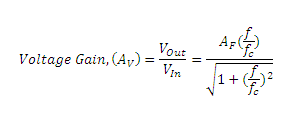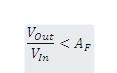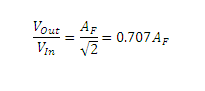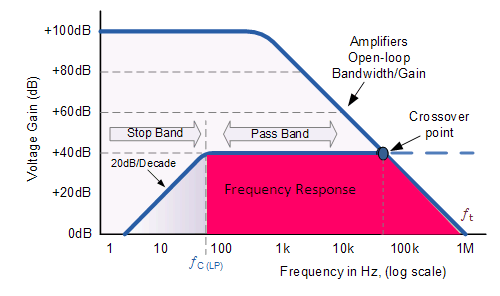# Active High Pass Filter Circuit Diagram and Operation

## Active High Pass Filter

Active High Pass Filter as its name implies, attenuates low frequencies and passes high frequency signals.

The basic operation of an Active High Pass Filter (HPF) is  same as that of a  passive high pass filter circuit, however the active high pass filter circuit has an operational amplifier or op-amp included in its design to provide amplification and gain control.

The simplest form of an active high pass filter is designed by connecting a standard inverting or non-inverting operational amplifier to the basic RC high pass passive filter circuit as shown below .

### Active High Pass Filter Circuit DiagramIt consists  of a passive filter section followed by a non-inverting operational amplifier.

The frequency response of the circuit is the same as that of the passive filter, except that the amplitude of the signal is increased by the gain of the amplifier.

for a non-inverting amplifier the magnitude of the voltage gain is given as a function of the feedback resistor ( R2 ) divided by its corresponding input resistor ( R1 ) i.e. 1 + R2/R1, the same as for the active low pass filter circuit.

Hence, the gain for an Active High Pass Filter is given as :Where:

A= Pass band Gain of the filter= ( 1 + R2/R1 )

ƒ = the Frequency of the Input Signal in Hz

ƒc = the Cut-off Frequency in Hz

The operation of a high pass active filter can be verified from the frequency gain equation above as:

1. At very low frequencies, ƒ < ƒc2. At the cut-off frequency, ƒ = ƒc3. At very high frequencies, ƒ > ƒcTherefore, the Active High Pass Filter has a gain AF that increases from 0 Hz to the low cutoff frequency  point, ƒC at 20dB/decade as the frequency increases.

At ƒC the gain is 0.707AF, and after ƒC all frequencies are pass band frequencies so the filter has a constant gain AF with the highest frequency being determined by the closed loop bandwidth of the op-amp.

### Frequency Response CurveThe maximum pass band frequency response of an active HPF is limited by the open-loop characteristics or bandwidth of the operational amplifier being used.

In our Operational amplifier tutorial we saw that the maximum frequency response of an op-amp is limited to the Gain/Bandwidth product or open loop voltage gain ( A V ) of the operational amplifier being used giving it a bandwidth limitation, where the closed loop response of the op amp intersects the open loop response.